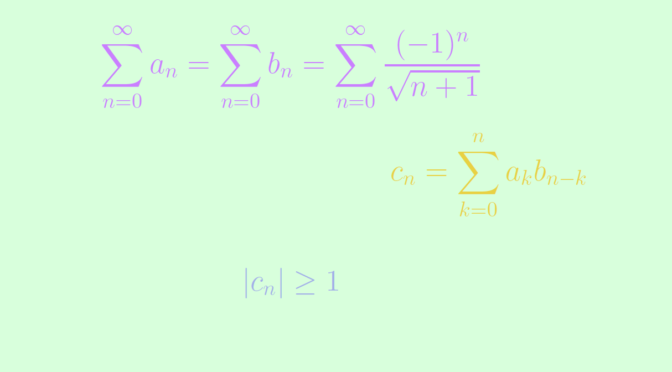# Counterexamples around the Cauchy product of real series

Let $$\sum_{n = 0}^\infty a_n, \sum_{n = 0}^\infty b_n$$ be two series of real numbers. The Cauchy product $$\sum_{n = 0}^\infty c_n$$ is the series defined by $c_n = \sum_{k=0}^n a_k b_{n-k}$ According to the theorem of Mertens, if $$\sum_{n = 0}^\infty a_n$$ converges to $$A$$, $$\sum_{n = 0}^\infty b_n$$ converges to $$B$$ and at least one of the two series is absolutely convergent, their Cauchy product converges to $$AB$$. This can be summarized by the equality $\left( \sum_{n = 0}^\infty a_n \right) \left( \sum_{n = 0}^\infty b_n \right) = \sum_{n = 0}^\infty c_n$

The assumption stating that at least one of the two series converges absolutely cannot be dropped as shown by the example $\sum_{n = 0}^\infty a_n = \sum_{n = 0}^\infty b_n = \sum_{n = 0}^\infty \frac{(-1)^n}{\sqrt{n+1}}$ Those series converge according to Leibniz test, as the sequence $$(1/\sqrt{n+1})$$ decreases monotonically to zero. However, the Cauchy product is defined by $c_n=\sum_{k=0}^n \frac{(-1)^k}{\sqrt{k+1}} \cdot \frac{(-1)^{n-k}}{\sqrt{n-k+1}} = (-1)^n \sum_{k=0}^n \frac{1}{\sqrt{(k+1)(n-k+1)}}$ As we have $$1 \le k+ 1 \le n+1$$ and $$1 \le n-k+ 1 \le n+1$$ for $$k = 0 \dots n$$, we get $$\frac{1}{\sqrt{(k+1)(n-k+1)}} \ge \frac{1}{n+1}$$ and therefore $$\vert c_n \vert \ge 1$$ proving that the Cauchy product of $$\sum_{n = 0}^\infty a_n$$ and $$\sum_{n = 0}^\infty b_n$$ diverges.

The Cauchy product may also converge while the initial series both diverge. Let’s consider $\begin{cases} (a_n) = (2, 2, 2^2, \dots, 2^n, \dots)\\ (b_n) = (-1, 1, 1, 1, \dots) \end{cases}$ The series $$\sum_{n = 0}^\infty a_n, \sum_{n = 0}^\infty b_n$$ diverge. Their Cauchy product is the series defined by $c_n=\begin{cases} -2 & \text{ for } n=0\\ 0 & \text{ for } n>0 \end{cases}$ which is convergent.